# Antinomy

(diff) ← Older revision | Latest revision (diff) | Newer revision → (diff)

A situation in which two mutual contradictory statements are demonstrated, each one having been deduced by means that are convincing from the point of view of the same theory.

As distinct from a sophism, which is a deliberately presented erroneous conclusion containing a masked error, an antinomy is usually an indication of deeper deficiencies of the theory in question. The discovery of an antinomy often leads to a thorough revision of the theory as a whole, attracts attention to new effects, and ultimately stimulates further studies. This feature of antinomies attracted the interest of philosophers ever since ancient times. One may recall, for example, the important role played by antinomies in the philosophy of E. Kant. A number of antinomies were studied in antiquity under the name aporium. Here, two famous antinomies named after Zeno of Elea (5th century B.C.) will be quoted.

"Achilles and the tortoiseAchilles and the tortoise" . This aporium deals with the contradiction involved in certain attributes of motion, and may be stated as follows. A runner (Achilles) is standing at a point, and a tortoise is standing at a point, 100 meters away from. At the same moment of time Achilles begins to run fromtowards, while the tortoise begins to move away fromin the direction away fromat a speed which is, say, 100 times less than that of Achilles. Experience teaches us that in such a situation Achilles will overtake the tortoise after a fairly short period of time. On the other hand, it is also possible to conclude that Achilles will never overtake the tortoise, and will not even reach the point. In fact, at the moment when Achilles has reached the midpoint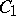of the distance, the tortoise will have moved away from, though only by a short distance. Next, Achilles will reach the midpoint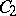of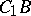, then the midpoint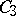of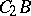, etc. Throughout that time the tortoise keeps moving away from. In order to reach, Achilles must have been present at each point in the infinite sequence of points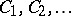. However, it is impossible to be present at an infinite number of points in a finite period of time; therefore, Achilles will never arrive at the pointand will never overtake the tortoise.

Paradoxes of this type are easily solved by methods of the modern mathematical theory of motion. A detailed analysis shows that such solutions materially depend on the truth of the so-called Archimedean axiom in the field of real numbers: For any real numbersthere exists a natural numbersuch that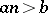. Nevertheless, the problem illustrated by the paradox is very real. It is possible to deny the convenience or the adequacy of the ordinary mathematical model as a description of real motion. In order to describe the concepts of infinitely small and infinitely large physical magnitudes, it was repeatedly attempted to construct a theory of real numbers in which the Archimedean axiom would not be valid. In any case, the theory of non-Archimedean ordered fields forms an essential part of modern algebra. Such non-Archimedean ordered fields — the non-standard real straight line — play a determining role in non-standard analysis.

The "sandpile paradoxsandpile paradox" may be stated as follows. Clearly, one grain of sand does not form a sandpile. Ifgrains of sand still do not form a sandpile, it follows that they will not form a pile after another grain of sand has been added. Accordingly, no number of grains of sand can form a sandpile.

This paradox can be solved in modern terms by saying that the method of complete mathematical induction cannot be applied to undefined volumes such as a "pile of sand grains" . In modern mathematics (second half of the 20th century) indefinite volumes are employed in the foundations of mathematics to establish the consistency of classical theories, and the properties of such concepts are studied by exact methods. It is possible to use methods of mathematical logic to realize a situation in which mathematical induction in its general form is not applicable to any natural series, even though very many ordinary properties of natural numbers remain valid in the process (the so-called non-standard model of arithmetic).

However, it is the antinomies involving unusual formulations of concepts which are undoubtedly of greatest interest to mathematics. These are the so-called logical and semantic antinomies (see also Skolem paradox).

Below three examples of logical antinomies are presented.

Russell's paradox (B. Russel, 1902), also independently discovered by E. Zermelo. Consider the following propertyof sets. Assume that a sethas the propertyif and only if it is not an element of itself. Propertyis valid for a very large majority of specific sets used in mathematical considerations. E.g., neither the set of natural numbers nor the set of all real numbers are elements of themselves. Consider the setsuch that its elements are exactly those setsthat have the property. Now consider whether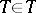oris true. Ifthen, by definition,has the property, i.e.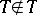. Thus, one must postulate. But this again results in a contradiction, since if,has the property, so that.

The paradox can be avoided by postulating that all that has been proved in this way is that there is no such set, i.e. that the propertydoes not characterize any set at all. However, this cannot be accepted, since it is natural to suppose, from the point of view of "naive" set theory, that any exactly-described propertyof objects defines a set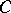of objects which possess this property. Russell's paradox is a strong blow to this natural concept. One must conclude that certain very simple properties must either be considered as imprecisely described, or else that there exist exactly-described properties which do not define a set. This in turn gives rise to several difficult questions. What are "exactly" and what are "inexactly" described properties? Which properties define a set and which do not? May not other properties which are extensively used in set theory also lead to contradictions and have to be discarded? Is it at least possible to reliably specify a domain which is adequately protected from paradoxes, and which is nevertheless sufficiently large to include conventional mathematical practice?

With the construction of exact logico-mathematical languages, the problem of the exact description of properties may be considered as being satisfactory solved. However, the problem of description of criteria establishing the class of properties defining a set is still a question that is far from solved. Even worse, modern results in axiomatic set theory indicate that there is no final solution to this problem. Russell's paradox made a great impression on his contemporaries since it concerned the very first stage in the study of set theory. Nevertheless, there exist various ways of avoiding paradoxes which, though hardly ultimate or natural, are very convenient in practice and throw light both on the nature of the paradox itself and on logical connections of other principles of set theory. The axiomatic approach to the fundamental principles of set theory (cf. Axiomatic set theory) proved particularly successful. Thus, the formal axiomatic system of Zermelo–Fraenkel is at the time of writing (1970's) a most useful axiomatic theory, which gives the most adequate description of the "non-paradoxical" part of the practice of classical set theory.

The paradox of the "village barber paradoxvillage barber" . This is a variant of Russell's paradox, formulated in the guise of an everyday-life situation. (A slightly-different form of this paradox is known as the Gonseth paradox.) It is the case of a village barber who shaves those and only those inhabitants of his village who do not shave themselves. Does the barber shave himself? A reasoning similar to that given in the context of Russell's paradox shows that he both shaves and does not shave himself. This difficulty is easily overcome if one concludes that there cannot be such a barber. The condition to be satisfied by the barber is self-contradictory and therefore cannot be satisfied. Admittedly, the problem raises the question of criteria of self-consistent properties but, unlike in Russell's paradox, this is by no means a burning problem. It refers to a real-life situation, and most such situations are never exactly formulated or reliably defined. Moreover, self-consistency is not the only criterion (nor probably even the most important one) of acceptability of real-life reasoning. The situation is altogether different in mathematical reasoning, which is supposed to be much more precisely defined and more reliable; self-consistency forms an important part of such a reasoning.

Cantor's paradox (G. Cantor, 1899). Letbe the set of all sets and let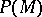be the set of all its subsets. It is clear from the definition ofthatis included in. On the other hand, in accordance with a well-known theorem of Cantor, the cardinality ofis larger than that of, and for this reasoncannot be a subset of. The conclusions which follow from Cantor's paradox are roughly the same as those following from Russell's paradox. In particular, it may be thought that Cantor's paradox proves that the setof all sets does not exist. It is interesting to note in this connection that there exist axiomatic systems of set theory, such as the well-known "New Foundations" system of W. Quine, which establish the existence of. Cantor's paradox can be avoided in the "New Foundations" owing to the fact that Cantor's cardinality theorem can be proved in this system only in a specific form, which is insufficient to "prove" the paradox. In general, the concepts of set theory required to construct Cantor's paradox are much more involved than those needed to construct Russell's paradox (such as the concept of a subset, the idea of a one-to-one correspondence, etc.).

A paradox which resembles Cantor's paradox is the so-called Burali–Forti paradox, which deals with the class of all ordinal numbers. The order type of this class must be larger than all the ordinals contained in it.

Antinomies of a somewhat different type, the so-called semantic antinomies, are also of major interest. As distinct from logical antinomies, semantic antinomies include terms such as "truth" , "falsehood" , "denotes" , "defines" and others. Otherwise, the difference between the two types of paradoxes, which was proposed by F. Ramsey in 1926, is largely arbitrary. Many semantic antinomies may be stated in a logical form and vice versa. It was noted by Ramsey that semantic antinomies cannot be constructed in ordinary logico-mathematical theories for the simple reason that such theories do not contain the semantic concepts which are required in the formulation of semantic antinomies. In this sense, semantic antinomies are "safer" than logical antinomies. It should be noted, however, that there are modern theories containing concepts for the formulation of certain semantic paradoxes, in particular Richard's paradoxes (but such paradoxes can always be avoided by using special devices). Below two well-known semantic paradoxes are stated.

Richard's paradox in the formulation of G.D.W. Berry (1906). Consider the set of natural numbers each one of which can be uniquely defined by a meaningful text containing not more than 1000 syllables. Obviously, the number of such texts is finite, since the collection of all texts with 1000 syllables is finite. Consider the smallest natural number that is not a member of the set defined above.

The above paragraph is a meaningful text, containing not more than 1000 syllables, which uniquely determines some natural number which, by definition, cannot be characterized by a set of this type. Obviously, this paradox can be avoided if this text is declared to be not meaningful (or to be a text which does not define a natural number), but in such a case, as before, there arise difficult problems concerning the criteria for a text to be meaningful, etc.

Eubulides' paradox (4th century B.C.). A person says: "What I am saying now is a lie" . Is this statement true or not? If it is true, its very meaning indicates that it is false. If it is false, it immediately follows that it is true. Many variants of this paradox are known — the liar's paradox, Epimenides' paradox, etc. The idea of this paradox forms the base of the proof of the well-known Gödel incompleteness theorem of formal axiomatic theories.

In general, analysis of such paradoxes stimulated a fundamental review of the views on the foundations of mathematics and the development of many modern ideas and methods of mathematical logic.

How to Cite This Entry:
Antinomy. Encyclopedia of Mathematics. URL: http://encyclopediaofmath.org/index.php?title=Antinomy&oldid=12051
This article was adapted from an original article by A.G. Dragalin (originator), which appeared in Encyclopedia of Mathematics - ISBN 1402006098. See original article